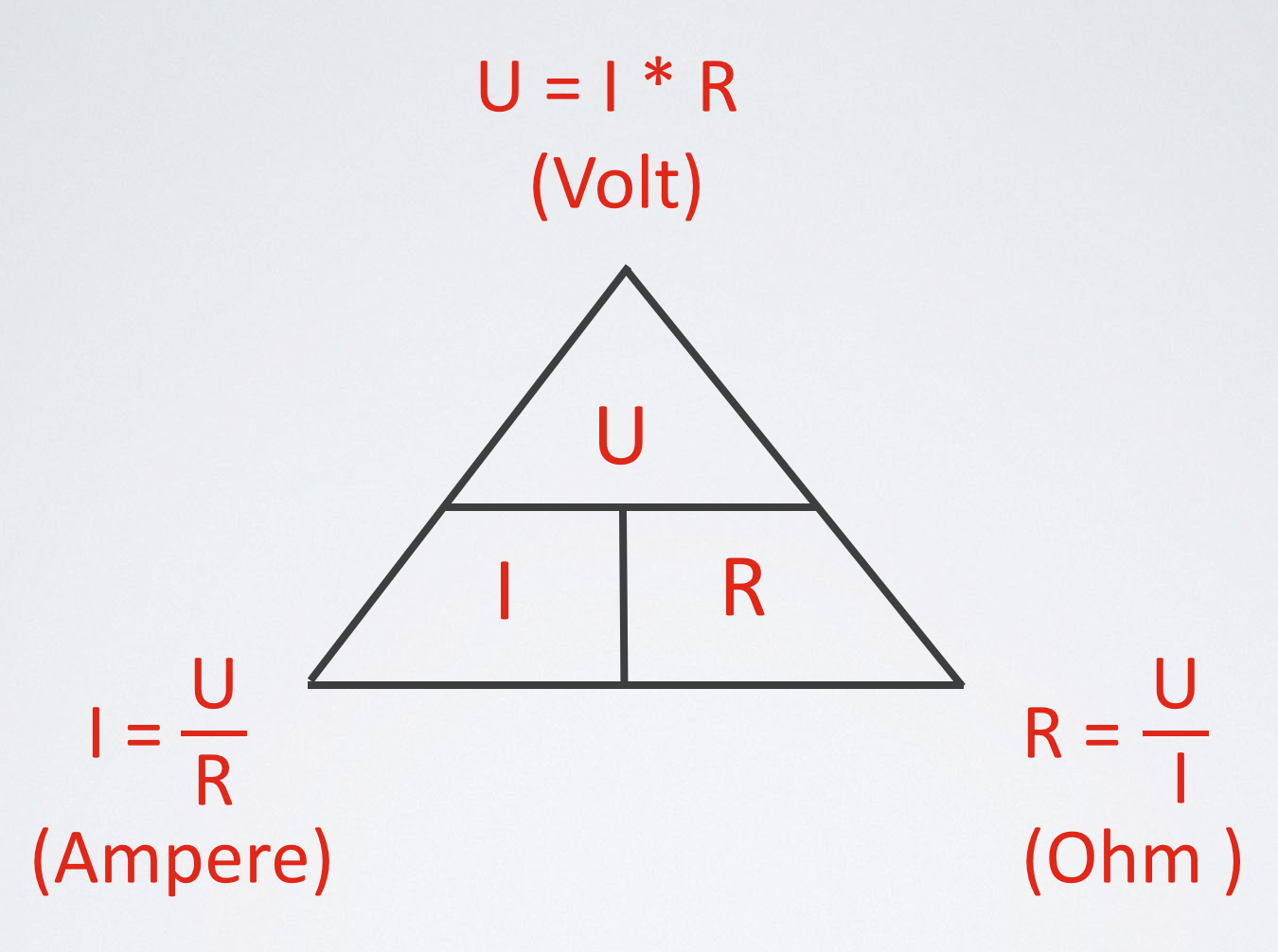Ohms Law.
If you want to understand electronics and build nice cool circuits, it's important to understand Ohms' law. It's the most fundamental law in Electronics. Check the small cartoon. This shows Ohms law ;-)

With a constant pressure (U; Voltage), the amount of current (I; Ampere) in a closed loop is determined by the total resistance (R; Ohm) of that loop.

In other words: U = I x RExample: if you connect a resistor of 1K (=1000Ohm) directly between the two poles of a 9V battery, the amount of current will be : I= U/R = 9/1000 = 9 mA = 0,009 A

Wiki: "Ohm's law states that the current through a conductor between two points is directly proportional to the potential difference across the two points. Introducing the constant of proportionality, the resistance, one arrives at the usual mathematical equation that describes this relationship"